Dave's Math Tables: Areas, Volumes, Surface Areas(Math | Geometry | AreasVolumes)

(pi =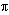= 3.141592...)

### Areas

square = a 2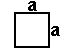rectangle = ab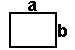parallelogram = bh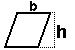trapezoid = h/2 (b1 + b2)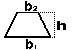circle = pi r 2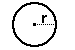ellipse = pi r1 r2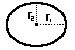triangle = (1/2) b h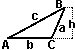equilateral triangle = [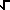(3)/2] a 2 =(3/4) a 2

triangle given SAS = (1/2) a b sin C

triangle given a,b,c =[s(s-a)(s-b)(s-c)] when s = (a+b+c)/2 (Heron's formula)

### Volumes

cube = a 3rectangular prism = a b c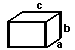irregular prism = b h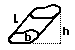cylinder = b h = pi r 2 hpyramid = (1/3) b h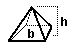cone = (1/3) b h = 1/3 pi r 2 h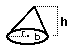sphere = (4/3) pi r 3ellipsoid = (4/3) pi r1 r2 r3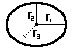### Surface Area

cube = 6 a 2prism:
(lateral area) = perimeter(b) L
(total area) = perimeter(b) L + 2bsphere = 4 pi r 2